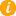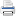# Math K-Plus

Math TestMath QuizQuiz Results• Option 2. Solve a calculator generated math problem. First press the NEW PROBLEM button. Next enter your answer, in to the calculator. Lastly, press the ADD button and then compare your answer with the calculator's.

The First Grade Multiplication Calculator has an extra feature. Not only is a complete numerical answer shown for each math problem, a visual answer is also shown; this helps students transition from thinking visually to thinking numerically.

Field Name Multiply Values
Multiplicand: You can enter only 1 digit
Multiplier: You can enter only 1 digit
×After learning First Grade Multiplication Calculator, you can move to the next step in multiplication math: Second Grade Multiplication Calculator.

+3
× +2
————

3
×  2
————

3
×  2
————
6

3
×  2
————
6